ChemTalk

# How to Find & Calculate Molar Mass## Core Concepts

In this tutorial, we will explain “what is molar mass”. You will learn how to find and calculate the molar mass for elements and molecules. If you enjoy this article, be sure to check out our other tutorials linked below.

## Vocabulary of Molar Mass

• Molar mass: The sum of atomic weight in a molecule. It is sometimes referred to as molecular weight. It is often abbreviated as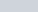.
• Compounds: Contains atoms of different elements that are combined at a fixed ratio.
• Ex. NaCl
• Molecules: Neutral group of atoms chemically bonded together.
• Ex. O3, NaCl
• Molecular formula: A way of presenting a molecule that shows the proportions of atoms.
• Ex. NH3 H2O2

## What is Molar Mass?

The definition of molar mass is simply the number of grams that one mole of a substance weighs. Another definition is the sum of the atomic weights of the atoms that make up a molecule. Both definitions will give you the same result, they are just in different units.

A “mole” of a substance is defined as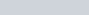atoms or molecules of that substance.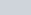is a one with 23 zeroes after it. That’s a heck of a lot of molecules, which is why the molecular mass of table salt (sodium chloride) is a respectable 58.44 grams per mole, quite a large handful.

The units of molar mass is grams per mole, often abbreviated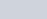.

## How to Calculate Molar Mass

Molar mass can be calculated by using the periodic table and following three simple steps. It should be noted that this number is an average and therefore may vary due to isotopic elements. Let’s look at some examples of calculating this value for some different molecules.

### Molar Mass of Ammonia NH3 – Step 1:

The first step for calculating molar mass is to identify all the elements in a given molecule and write their atomic masses using the periodic table. The atomic mass is equal to the atomic number which is listed below the element symbol. For example, if we are trying calculate for ammonia (NH3), then we need to find the atomic masses for nitrogen and hydrogen. Using the periodic table, we should get:

• Nitrogen: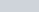• Hydrogen: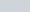### Step 2:

The second step is to determine how much of each element is present in the compound. According to the molecular formula (NH3), there is one nitrogen and three hydrogens present. So, now we will multiply these numbers by their corresponding atomic masses. It should look like this:

• Nitrogen: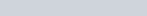• Hydrogen: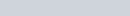### Step 3:

The last and final step is to add up the two products we got from multiplying. For ammonia, we should therefore get a molecular mass of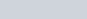. Moreover, this means that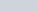of NH3 is equal to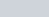of NH3.

Nitrogen and hydrogen: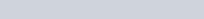## Carbon Dioxide (CO2) Example Problem

• Carbon:• Oxygen: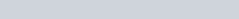## Water (H2O) Example Problem

• Hydrogen: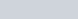• Oxygen: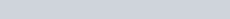## Molar Mass Practice Problems

Problem 1

You have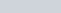of some unknown transition metal which weighs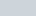. What is the identity of this metal?

Problem 2

The organic molecule cyclobutadiene (C4H6) combines with propenoic acid (C3H4O2) to form a molecule with the molecular formula of C7H10O2. Later, after heating, the molecule releases a molecule of carbon dioxide (CO2). What is a molar mass of the final product?

## Molar Mass Practice Problem Solutions

1: Manganese (Mn) (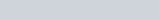)

2:(C6H10)